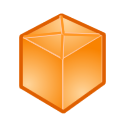﻿ LitDev API

LitDev Extension API

 LD3DView LDArray LDBits LDBlueTooth LDCall LDChart LDClient LDClipboard LDColours LDCommPort LDController LDControls LDCursors LDDataBase LDDateTime LDDebug LDDialogs LDDictionary LDEffect LDEmail LDEncryption LDEvents LDFastArray LDFastShapes LDFigures LDFile LDFocus LDftp LDGraph LDGraphicsWindow LDHID LDIcon LDImage LDInline LDIOWarrior LDList LDLogic LDMath LDMathX LDMatrix LDMusic LDNetwork LDPhysics LDProcess LDQueue LDRegex LDResources LDScrollBars LDSearch LDServer LDSettings LDShapes LDShell LDSort LDSound LDSpeech LDStatistics LDStopwatch LDText LDTextWindow LDTimer LDTranslate LDUnits LDUtilities LDVector LDWaveForm LDWebCam LDWindows LDxml LDZip

LDMathTrig functions with degrees.
Other assorted maths functions and calculations.

 ArcCosArcSinArcTanBase2DecimalConvert2CartesianConvert2RadialCosCoshDecimal2BaseEEvaluateEvaluate2Evaluate3ExpFixDecimalFixSigFigMaxNumberMinNumberRandomNumberSeedRotateSinSinhTanTanhTruncateArcCos(cos)ArcCos in degrees.
cos The Cos of the angle.
returns The angle in degrees.

ArcSin(sin)ArcSin in degrees.
sin The Sin of the angle.
returns The angle in degrees.

ArcTan(tan)ArcTan in degrees.
tan The Tan of the angle.
returns The angle in degrees.

Base2Decimal(number,Base)Convert a base number to a decimal integer.
number The base number to convert (non negative).
Base The base to convert from (2 binary) (8 octal) (16 hex) or other bases up to 36.
returns The number as a decimal integer or "FAILED".

Convert2Cartesian(x1,y1,dist,angle)Calculate the coordinates of a point 2, from point 1 and the distance and angle to point 2.
x1 The X coordinate of point 1.
y1 The Y coordinate of point 1.
dist The distance to point 2.
angle The angle to point 2 in degrees.
returns A 2 element array with the coordinates of point 2.

Convert2Radial(x1,y1,x2,y2)Calculate the distance and angle between two points.
x1 The X coordinate of point 1.
y1 The Y coordinate of point 1.
x2 The X coordinate of point 2.
y2 The Y coordinate of point 2.
returns A 2 element array with the distance and the angle in degrees from point 1 to point 2.

Cos(angle)Cos of an angle in degrees.
angle The angle in degrees.
returns The Cos of the angle.

Cosh(angle)Hyperbolic cosine.
angle Angle in radians.
returns Hyperbolic cosine.

Decimal2Base(number,Base)Convert a decimal integer to another base.
number The decimal integer to convert (non negative).
Base The base to convert to (2 binary) (8 octal) (16 hex) or other bases to 36.
returns The number in the requested base or "FAILED".

ENatural logarithm base.

Evaluate(expression)Evaluate a string expression to a number or boolean (if possible).
The JScript command 'eval' is used and may therefore allow more complex JScript manipulations (also see LDInline).
The TextWindow should be visible prior to using this method if later use of the TextWindow is required.

expression The expression to evaluate, e.g. "(9/6) + 3" or "2.1 > 1.5".
returns The evaluated result.

Evaluate2(expression)Evaluate a string expression to a number (if possible).
An alternative to Evaluate that behaves nicely with the TextWindow.

expression The expression to evaluate, e.g. "1e6 + 6/4".
returns The evaluated result.

Evaluate3(expression)Evaluate a string expression to a boolean "True" or "False" (if possible).
An alternative to Evaluate that behaves nicely with the TextWindow.

expression The expression to evaluate to a boolean, e.g. "21.3 > 16".
returns The evaluated result ("True" or "False").

Exp(value)Exponential of a number e^x.
value The value to raise e to the power of.
returns e^x

FixDecimal(number,digits)Round a number to a fixed number of decimal places. Additional training decimal 0s are added if required.
number The number to change.
digits The number of decimal places.
returns The modified number.

FixSigFig(number,digits)Round a number to a fixed number of significant figures.
number The number to change.
digits The number of significant figures.
returns The modified number.

MaxNumberThe maximum number that Small Basic can handle.

MinNumberThe minimum number that Small Basic can handle.

RandomNumberSeedSet the seed for random numbers. The seed should be an integer number and be set before Math.GetRandomNumber is called.
The random number sequence will be initialised by the seed and can be used for testing when a repeatable sequence of random numbers is required.

Rotate(x1,y1,x2,y2,angle)Rotate point 2 clockwise about point 1.
x1 The X coordinate of point 1.
y1 The Y coordinate of point 1.
x2 The X coordinate of point 2.
y2 The Y coordinate of point 2.
angle The angle to rotate point 2 around point 1 in degrees.
returns A 2 element array with the coordinates of the rotated point 2.

Sin(angle)Sin of an angle in degrees.
angle The angle in degrees.
returns The Sin of the angle.

Sinh(angle)Hyperbolic sine.
angle Angle in radians.
returns Hyperbolic sine.

Tan(angle)Tan of an angle in degrees.
angle The angle in degrees.
returns The Tan of the angle.

Tanh(angle)Hyperbolic tangent.
angle Angle in radians.
returns Hyperbolic tangent.

Truncate(value)Get the integral part of a number.
value The number to truncate.
returns The integral part of the number (removing decimal fraction).